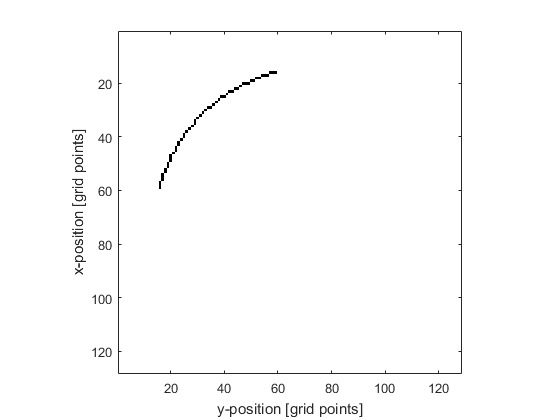# makeArc

Create a binary map of an arc within a 2D grid.

## Syntax

```arc = makeArc(grid_size, arc_pos, radius, diameter, focus_pos)
arc = makeArc(grid_size, arc_pos, radius, diameter, focus_pos, ...)
```

## Description

`makeArc` creates a binary map of an arc within a two-dimensional grid. The arc position is denoted by 1's in the matrix with 0's elsewhere. The arc is found using the mid-point circle algorithm, and is simply connected so that any grid point on the arc will have at most 2 neighbours.

The midpoint of the arc is set by `arc_pos`. The orientation of the arc is set by `focus_pos`, which corresponds to any point on the axis of the arc (note, this must not be equal to `arc_pos`). It is assumed that the arc angle is equal to or less than pi radians. If the radius is set to `inf`, a line is generated.

## Examples

```% define parameters
grid_size = [128, 128];
arc_pos   = [32, 32];
diameter  = 61;
focus_pos = grid_size;

% create arc
arc = makeArc(grid_size, arc_pos, radius, diameter, focus_pos, 'Plot', true);
```## Inputs

 `grid_size` size of the 2D grid given as a three element vector [Nx, Ny] [grid points] `bowl_pos` midpoint of the arc given as a two element vector [ax, ay] [grid points] `radius` radius of curvature of the arc [grid points] `diameter` aperture diameter (length of line connecting arc endpoints) [grid points] `focus_pos` any point on the beam axis of the arc given as a two element vector [fx, fy] [grid points]

## Optional Inputs

Optional 'string', value pairs that may be used to modify the default computational settings.

Input Valid Settings Default Description
`'Plot'` (Boolean scalar) `false` Boolean controlling whether the arc is plotted using `imagesc`.

## Outputs

 `arcs` 2D binary map of arcs `arc` 2D labelled matrix of arcs

`makeBowl`, `makeLine`, `makeMultiArc`